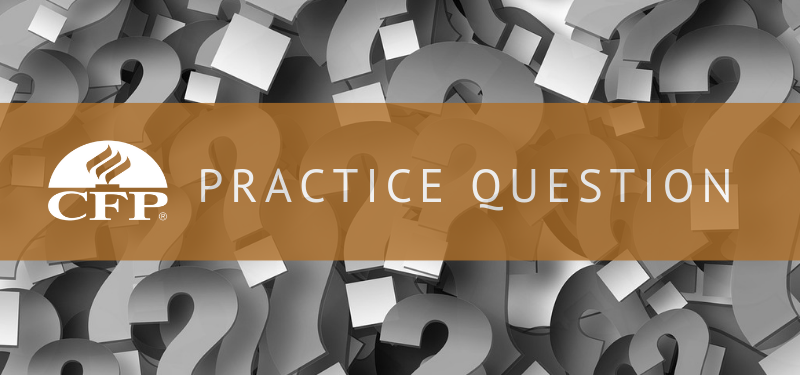# CFP® Practice Question: Which Portfolio Would You Choose?Portfolio X has a weighted beta coefficient of 1.5, and Portfolio Y has a weighted beta coefficient of .8. Both portfolios are expected to earn the same weighted-average expected return. With these assumptions, which of the following statements is correct?

1. An investor should choose Portfolio X because of its higher beta.
2. An investor should choose Portfolio Y because it carries less risk for the given expected return.
3. An investor may be indifferent between the two since the expected return is the same.
4. The information given is not helpful in making a choice.